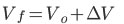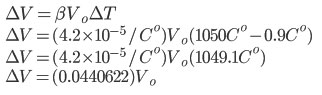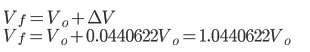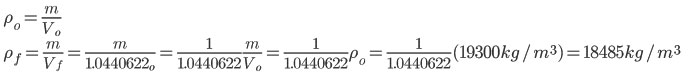SEARCH HOMEMath Central Quandaries & QueriesQuestion from florence, a student: Hi- Please help me to apply the formula for this problem. The coefficient of volumetric for gold is 4.20 X 10-5 C degrees. The density of gold is 19,300 kg/m3 at 0.9 C degrees. What is the density of gold at 1050 degrees C. Could you please explain how to get the solution of 18,500 kg/m3 Thank you for your help FlorenceHi Florence,

We need to consider the expansion of the gold as it heats up to determine the density at the higher temperature.The final volume will be the initial volume plus how much the volume has expanded.To find the change in volume we multiply the coefficient of thermal expansion by the initial volume and change in temperature. The coefficient of thermal volume of expansion for gold is β or in our case 4.2×10-5/Co.Now that we know how much the gold has expanded relative to the original volume we can determine the final volume.

Since density is a ratio of the mass to volume, we can find the final density in terms of initial density:Our original density only had 3 significant figures so the answer would be 18500 kg/m3

Hope this helps,

JaniceMath Central is supported by the University of Regina and The Pacific Institute for the Mathematical Sciences.